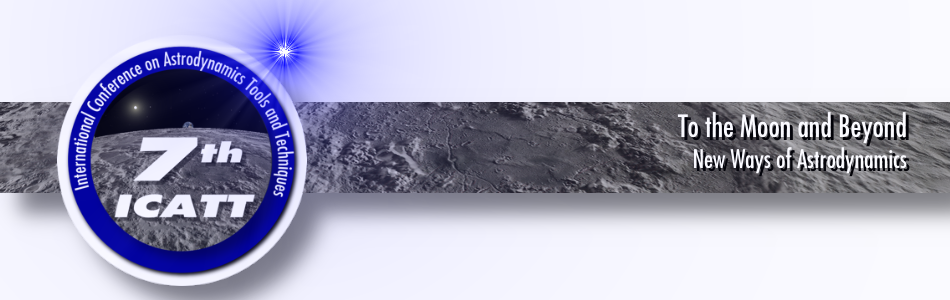#7th International Conference on Astrodynamics Tools and Techniques (ICATT)

6-9 November 2018
DLR Oberpfaffenhofen, Germany
Europe/Amsterdam timezone

## OPEN SOURCE ORBIT DETERMINATION WITH SEMI-ANALYTICAL THEORY

7 Nov 2018, 14:30
30m
Single 107

### Single 107

Building 135
Oral presentation at the conference 11: Open Source Tools and Smart Computing

### Speaker

Luc Maisonobe (CS SI)

### Description

Space objects catalog maintenance demands an accurate and fast orbit determination (OD) process to cope with the ever increasing number of observed space objects. The development of new methods, that answer the two previous problems, becomes essential.

Presented as an alternative to numerical and analytical methods, the Draper Semi-analytical Satellite Theory (DSST) is an orbit propagator based on a semi-analytical theory allowing to preserve the accuracy of a numerical method while providing the speed of an analytical method. This propagator allows computing the mean elements and the short-period effects separately. We reproduced this architecture at the OD process level in order to be able to return, as desired, the mean elements or the osculating elements. Two major cases of use are thus possible: fast OD for big space objects catalog maintenance and mean elements OD for station keeping issues.

This paper presents the different steps of development of the DSST OD included in the Orekit open-source library. Integrating an orbit propagator into an OD process can be a difficult procedure. Computing and validating derivatives is a critical step, especially with the DSST whose equations are very complex. To cope with this constraint, we used the automatic differentiation (AD) technique. AD has been developed as a mathematical tool to avoid the calculations of the derivatives of long equations. This is equivalent to calculating the derivatives by applying chain rule without expressing the analytical formulas. Thus, AD allows a simpler computation of the derivatives and a simpler validation. AD is also used in Orekit for the propagation of the uncertainties using the Taylor algebra.

Existing OD applications based on semi-analytical theories calculate only the derivatives of the mean elements. However, for higher accuracy or if the force models require further development, adding short-period derivatives improves the results. Therefore, our study implemented the full contribution of the short-period derivatives, for all the force models, in the OD process. Nevertheless, it is still possible to choose between using the mean elements or the osculating elements derivatives for the OD.

This paper will present how the Jacobians of the mean rates are calculated by AD into the DSST-specific force models. It will also present the computation of the state transition matrices during propagation. Both mean elements and short-periodic derivatives are developed. The performance of the DSST OD is demonstrated under Lageos2 and GPS orbit determination conditions.

Keywords: Orbit determination, Automatic differentiation, Semi-analytical theory, DSST, Open-source, Orekit.